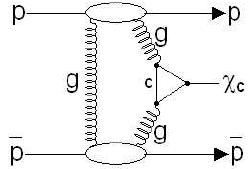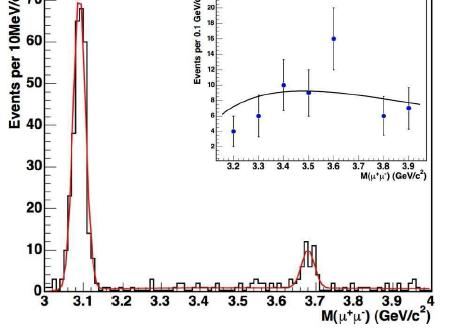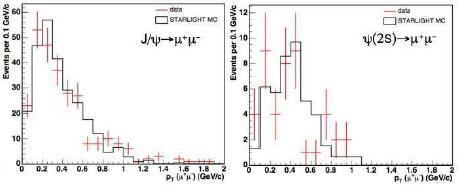## Three exquisite exclusive charmonium signalsDecember 29, 2008

Posted by dorigo in news, physics, science.
Tags: , , ,

It is my pleasure today to report on an extremely interesting measurement recently produced by the CDF experiment, one which is dear to me for several reasons.

First of all, the measurement involves the study of charmonium -a fascinating system made by a charm-anticharm quark pair, the two quarks orbiting around each other for a tiny fraction of a second before annihilating. Charmonium -in the form of the$J/\psi$ particle- is the state of matter discovered in November 1974 by Nobel Laureates Samuel Ting (at Brookhaven) and Burton Richter (at SLAC), and which immediately confirmed the reality of quarks -entities whose reality had kept many brilliant minds skeptical during the previous decade. The$J/\psi$ and the other charmonium states have been since then a gold mine for the study of Quantum Chromodynamics, of B hadron phenomenology (through the easy-to-spot decays of the type$B \to J/\psi X$), and an invaluable calibration tool for particle trackers. Charmonium may one day be even used with profit to measure with high precision the top-quark mass at the LHC!

Second, the study focuses on what is called “exclusive production”, a spectacular signature at hadron colliders: it involves the production of the particle without the disintegration of the projectiles, through a colourless exchange. Basically, the charmonium meson appears out of nothing, and in the company of exactly nothing else. It is as if by a stroke of a magic wand, a spark is exchanged between a proton and an antiproton coming close together, and a single particle is created. Those of you who know what kind of a mess is routinely yielded by proton-antiproton collisions at 2 TeV of energy in the core of CDF and D0 cannot but join my awe for the very concept of clean single-body production.

Third, exclusive production processes are quite rare in hadron collisions, and they are extremely interesting because they may, one day, provide the means by which we can access a direct measurement of the quantum numbers of the Higgs boson at the LHC. Also, their theoretical calculation is not on very solid ground, so a measurement is of great help. This one by CDF is the first ever on exclusive charmonium in hadron-hadron collisions.

Some notes on the production mechanisms

Before I come to describe the measurement details, let me present you with two Feynman diagrams of the simplest reactions responsible for the exclusive creation of a charmonium system. As I said above, the exchange between proton and antiproton is colourless: this, while still being a QCD interaction! But how is it possible for quantum chromodynamics to mediate a colourless reaction ? We know that the carrier of the strong force is the gluon, a bi-coloured object. On the other hand, if a proton emits or absorbs a gluon, it will never keep intact: it will become coloured, and explode like a firework. Unless…Unless a second gluon immediately comes to the rescue, restoring the neutrality of the proton! This is what is needed for a strong interaction to produce a charmonium state: two gluons are emitted and absorbed by the proton and the antiproton, and the pair can thus leave the interaction point, slightly rattled but unharmed. The first pair of gluons meets halfways, creating a charm-quark loop. The loop then “closes” into a$\chi_c$ meson, a charmonium state with the right quantum numbers. This is the process shown on the right.

Notice that a two-gluon exchange cannot give rise to a$J/\psi$ meson. This is because of the invariance of strong interactions for the operation called “charge conjugation”. A discussion of the invariance properties of particle reactions under the operation of exchanging every particle with its antiparticle would take us too far, and is the proper subject of another post. I will just say here that the reaction is impossible: in quantum mechanics, one says that the “transition amplitude vanishes” for a two-gluon state converting into a vector particle.

Not so for the$\chi_c$ though, since that particle has zero angular momentum: it is not a vector, but a pseudoscalar meson. That is why we have examined$\chi_c$ production first. But exclusive$J/\psi$ production does occur anyway.

How, however, if we are to keep the two incoming baryons intact? Let us think at the problem from the point of view of the$J/\psi$ then. This particle is known to be quite slow to decay into hadrons. In fact, it has a lifetime much longer than similar hadronic states. So much so, in fact, that the very suppressed electromagnetic decay into electron or muon pairs gets a sizable share of the probability. An electromagnetic process competing with a strong process ? This is very weird: we know that the electromagnetic decay must have a rate proportional to the coupling constant$\alpha$, which is fifty times smaller than the strong coupling constant$\alpha_s$ for such low-energy phenomena. However, how is quantum chromodynamics going to disintegrate a$J/\psi$ ?

Phase one is simple: the charm and the anticharm get together in the same point of space, annihilating. Phase two is the emission of gluons: not a single one though, because the$J/\psi$ is colourless! And not just two, because of the quantum mechanical selection rule concerning C-parity we mentioned above! The minimum number of gluons is three. That means that we have to take the third power of$\alpha_s$ when computing the decay rate through QCD interactions, and so electromagnetic processes become competitive.

In reality, these three-gluon exchanges are even more suppressed than they ought to be. This is encoded in what is called “OZI rule”, by the name of Okubo, Zweig, and Iizuka: at any order of the strong coupling constant$\alpha_s$, “disconnected” strong interaction processes -ones that have no fermion lines propagating from the initial to the final state, but only gluons- occur with smaller frequency. This is a consequence of the variability of$\alpha_s$ with the energy of the gluon emission: in disconnected diagrams gluons have to carry an energy at least equal to the mass of the particles they are going to materialize in the final state, and the corresponding value of the coupling constant is therefore evaluated at a higher energy, and is thus much smaller than the one involved in the emission of softer gluons in non-disconnected diagrams. Confused ? Not a problem, forget the argument and let us return to$J/\psi$ production.

The above discussion just meant to show that we need three gluons to produce one$J/\psi$, since we now know that one such particle can decay to no less than three gluons. Now, let us take the protons -the projectiles producing the reaction- in the equation. Who is going to provide two gluons, and who is going to provide only one ? I can picture the proton and antiproton starting a bitter argument on this issue. The one who provides a single gluon is a dead baryon, since it will get its colourlessness unbalanced, and it will disgregate. This is bad, because the production process is not exclusive any longer if, together with a$J/\psi$ meson, we get additional hadrons.How then can we produce a vector charmonium particle in an exclusive fashion ? Electrodynamics comes to the rescue. One of the two projectiles yields a pair of gluons, and the other emits a photon. Both can remain intact this way, and angular momentum does not object. The process is shown on the right: as you see, the charm-anticharm pair becomes a colourless one after the second gluon is exchanged, and a$J/\psi$ (or a$\psi(2S)$ meson, which has the same properties except angular momentum) arises.

The CDF search

Now that we know how proton-antiproton collisions are expected to yield exclusive charmonium, we may look for it more meaningfully.

The search uses an integrated luminosity of 1.5 inverse femtobarns of proton-antiproton collisions, recorded by the CDF experiment during Run II. The trigger used to collect exclusive production candidates selects data with two muon tracks of opposite charge, with no energy recorded in the BSC1 system, a set of scintillation counters covering the rapidity intervals$5.4 < |\eta| < 5.9$. Such a requirement excludes data which may be similar kinematically to real exclusive production, but feature a few hadrons produced almost parallel to the proton-antiproton direction.

Offline, events are excluded if they contain any other detected particle but the two muons. However, the search for$\chi_c$ allows one electromagnetic energy cluster in the calorimeter, signalling the potential photon emitted along with two muons in the$\chi_c \to \mu^+ \mu^- \gamma$ decay.

After those cuts, the only potential background to address is cosmic-ray muons that cross the detector, leaving a long track that may be fit as a pair of opposite-charge muons if it crosses the detector in proximity of the beam. These events are rejected by requiring that the two muons have an azimuthal opening angle smaller than 3.0 radians, and rejecting ones that have timing information incompatible with the instant when the proton and antiproton bunches cross in the detector.

The invariant mass of the 402 events surviving the selection is histogrammed in the graph below, if it is in the 3.0 – 4.0 GeV range (ignore the inset, which shows a fit to the background). A clear peak of$J/\psi$ and$\psi(2S)$ candidates is apparent on a flat background of non-resonant QED dimuon pairs; the set of events in the$J/\psi$ peak which contain one additional photon candidate make the$\chi_c$ signal (65 events).The calculation of efficiencies is complicated by the fact that the exclusivity requirements remove bunch crossings which produced more than a single proton-antiproton interaction; this is a function of the number of particles in the bunches. After all is accounted properly, CDF can however determine the cross-section for charmonium production in exclusive processes with a good accuracy. The results are as follows:

•$\frac{d \sigma}{dy} (y=0) = 3.92 \pm 0.62 nb$ for$J/\psi$
•$\frac {d \sigma}{dy} (y=0) = 0.54 \pm 0.15 nb$ for$\psi(2S)$
•$\frac {d \sigma}{dy} (y=0) = 75 \pm 14 nb$ for$\chi_c$

The above results are in agreement with theoretical calculations, which have very large uncertainties. This measurement was long overdue: it is a very important input for theoretical calculations of exclusive processes.

The kinematical characteristics of the resulting$J/\psi$ and$\psi(2S)$ decay signals are studied by comparing data with a Monte Carlo simulation of photoproduction, finding good agreement. Below are shown the transverse momentum distributions of the resonances in the data (red points), compared to simulation (black histograms).In conclusion, I am happy to see these very intriguing signals appear in CDF data, and I congratulate with the main authors of the analysis, Long Zhang, Mike Albrow, and James Pinfold! You can find more information on the analysis in the public web page of the result.